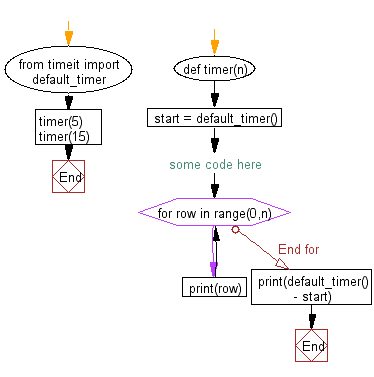﻿ Python: Calculate the time runs of a program - w3resource# Python: Calculate the time runs of a program

## Python Basic: Exercise-133 with Solution

Write a Python program to calculate the time runs (difference between start and current time)of a program.

Sample Solution:-

Python Code:

``````from timeit import default_timer
def timer(n):
start = default_timer()
# some code here
for row in range(0,n):
print(row)
print(default_timer() - start)

timer(5)
timer(15)
```
```

Sample Output:

```0
1
2
3
4
2.6107000849151518e-05
0
1
2
3
4
5
6
7
8
9
10
11
12
13
14
4.1371999941475224e-05
```

Flowchart:Python Code Editor:

Have another way to solve this solution? Contribute your code (and comments) through Disqus.

What is the difficulty level of this exercise?

Test your Python skills with w3resource's quiz

﻿

## Python: Tips of the Day

List comprehension:

```>>> m = [x ** 2 for x in range(5)]
>>> m
[0, 1, 4, 9, 16]
```